# Semi-group of holomorphic mappings

Non-linear semi-group theory is not only of intrinsic interest, but is also important in the study of evolution problems (cf. also Evolution equation). In recent years (as of 2000) many developments have occurred, in particular in the area of non-expansive semi-groups in Banach spaces. As a rule, such semi-groups are generated by accretive operators (cf. also Accretive mapping) and can be viewed as non-linear analogues of the classical linear contraction semi-groups (cf. also Contraction semi-group). Another class of non-linear semi-groups consists of the semi-groups generated by holomorphic mappings. Such semi-groups appear in several diverse fields, including, for example, the theory of Markov stochastic branching processes, Krein spaces, the geometry of complex Banach spaces, control theory, and optimization. These semi-groups can be considered natural non-linear analogues of semi-groups generated by bounded linear operators (cf. also Semi-group of operators). These two distinct classes of non-linear semi-groups are related by the fact that holomorphic self-mappings are non-expansive with respect to Schwarz–Pick pseudo-metrics (see, for example, [a8], [a7], [a6]).

Recall that a function $h$ defined on a domain (open connected subset) $\textbf{D}$ in a complex Banach space $X$ and with values in a complex Banach space is said to be holomorphic in $\textbf{D}$ if for each $x\in\textbf{D}$ the Fréchet derivative of $h$ at $x$ (denoted by $Dh(x)$ or $h'(x)$) exists as a bounded complex-linear mapping of $X$ into the Banach space containing the values of $h$. (Cf. also Banach space of analytic functions with infinite-dimensional domains.)

If $\textbf{D}$ and $\Omega$ are domains in complex Banach spaces $X$ and $Y$, respectively, then the set of holomorphic mappings from $\textbf{D}$ into $\Omega$ is denoted by $\text{Hol}(\textbf{D},\Omega)$. The notation $\text{Hol}(\textbf{D})$ is used to denote the set $\text{Hol}(\textbf{D},\textbf{D})$ of holomorphic self-mappings of $\textbf{D}$.

A family $\{S(t)\subset\text{Hol}(\textbf{D}\}$, where $t\in(0,T)$, $T>0$, is called a (one-parameter) continuous semi-group if

\begin{equation}S(s+t)=S(s)\circ S(t),s,t,s+t\in(0,T),\end{equation}

and

\begin{equation}\lim_{t\to 0^{+}}S(t)(x)=x,x\in D,\end{equation}

where the limit is taken with respect to the strong topology of $X$.

## Differentiability of semi-groups with respect to the parameter.

Let $\{S(t)\}$, $t\in(0,T)$, be a continuous semi-group defined on $\textbf{D}$. If the strong limit

\begin{equation}g(x)=\lim_{t\to 0^{+}}\frac{1}{t}(x-S(t)(x))\end{equation}

exists for each $x\in \textbf{D}$, then $g\in\text{Hol}(\textbf{D},X)$ is called the (infinitesimal) generator of the semi-group $\{S(t)\}$. In this case the semi-group $\{S(t)\}$, $t\in(0,T)$, is said to be differentiable (or generated).

For the finite-dimensional case, M. Abate proved in [a2] that each continuous semi-group of holomorphic mappings is everywhere differentiable with respect to its parameter, i.e., it is generated by a holomorphic mapping. In addition, he established a criterion for a holomorphic mapping to be a generator of a one-parameter semi-group. Earlier, for the one-dimensional case, similar facts were presented by E. Berkson and H. Porta in their study [a4] of linear $C_0$-semi-groups of composition operators on Hardy spaces. E. Vesentini investigated semi-groups of fractional-linear transformations that are isometries with respect to the infinitesimal hyperbolic metric on the unit ball of a Banach space [a18]. He used this approach to study several important problems in the theory of linear operators on indefinite metric spaces. Note that, generally speaking, such semi-groups are not everywhere differentiable in the infinite-dimensional case.

In fact, it can be shown (see for example, [a14]), that a continuous semi-group $S(t)$ of holomorphic self-mappings of a domain $\textbf{D}$ in $X$ is generated if and only if the convergence in (2) is locally uniform on $\textbf{D}$ (cf. also Uniform convergence).

Moreover, if $\textbf{D}$ is hyperbolic (in particular, bounded; cf. also Hyperbolic metric), then $S(t)$ can be continuously extended to all of $\textbf{R}^+=[0,\infty)$ as the solution of the Cauchy problem

\begin{equation}\begin{cases}\frac{\partial u(t,x)}{\partial t}+g(u(t,x))=0,\\u(0,x)=x,\end{cases}\end{equation}

where $x\in \textbf{D}$ and $t\in \textbf{R}^+$, i.e.,

\begin{equation}u(t,x)=S(t)x,x\in \textbf{D},t\in[0,\infty)\end{equation}

(see, for example, [a12], [a13]). Thus, there is a one-to-one correspondence between locally uniformly continuous semi-groups and their generators. If $S(t)$ has a continuous extension to all of $\textbf{R}=(-\infty,\infty)$, then it is actually a one-parameter group of automorphisms of $\textbf{D}$.

## Exponential and product formulas.

A holomorphic vector field

\begin{equation}T_g=g(x)\frac{\partial}{\partial x}\end{equation}

on a domain $\textbf{D}$ is determined by a holomorphic mapping $g\in\text{Hol}(\textbf{D},X)$ and can be regarded as a linear operator $T$ mapping $\text{Hol}(\textbf{D},X)$ into itself, where $T_gf\in\text{Hol}(\textbf{D},X)$ is defined by

\begin{equation}(T_gf)(x)=Df(x)g(x),x\in\textbf{D}\end{equation}

The set of all holomorphic vector fields on $\textbf{D}$ is a Lie algebra under the commutator bracket

\begin{equation}[T_g,T_h]=\bigg[g(x)\frac{\partial}{\partial x}, h(x)\frac{\partial}{\partial x}\bigg]:=\\=(Dg(x)h(x)-Dh(x)g(x))\frac{\partial}{\partial x}\end{equation}

(see, for example, [a9], [a15], [a6]).

Furthermore, each vector field (6) is locally integrable in the following sense: for each $x\in\textbf{D}$ there exist a neighbourhood $\Omega$ of $x$ and a $\delta>0$ such that the Cauchy problem (4) has a unique solution $\{u(t,x)\}\subset\textbf{D}$ defined on the set $\{|t|<\delta\}\times\Omega\subset\textbf{R}\times\textbf{D}$.

A holomorphic vector field $T_g$ defined by (6) and (7) is said to be (right) semi-complete (respectively, complete) on $\textbf{D}$ if the solution of the Cauchy problem (4) is well-defined on all of $\textbf{R}^+\times\textbf{D}$ (respectively, $\textbf{R}\times\textbf{D}$), where $\textbf{R}^+=[0,\infty)$ (respectively, $\textbf{R}=(-\infty,\infty)$).

Thus, if $\textbf{D}$ is hyperbolic, then $T_g$ is semi-complete (respectively, complete) if and only if $g$ is the generator of a one-parameter continuous semi-group (respectively, group).

On the other hand, if $\textbf{D}$ is bounded and $\widetilde{\text{Hol}}(\textbf{D},X)$ is the subspace of $\text{Hol}(\textbf{D},X)$ consisting of all $f\in\text{Hol}(\textbf{D},X)$ that are bounded on each ball strictly inside $\textbf{D}$, then a semi-group (group) $\{S(t)\}$, $t\in \textbf{R}^+$ (respectively, $t\in\textbf{R}$), induces a linear semi-group (group) $\{\mathcal{L}(t)\}$ of linear mappings $\mathcal{L}(t):\widetilde{\text{Hol}}(\textbf{D},X)\to\widetilde{\text{Hol}}(\textbf{D},X)$, defined by

\begin{equation}(\mathcal{L}(t)f)(x):=f(S(t)x),\end{equation}

where $t\in\textbf{R}^+$ ($t\in\textbf{R}$) and $x\in\textbf{D}$.

This semi-group is called the semi-group of composition operators on $\widetilde{\text{Hol}}(\textbf{D},X)$. If $\{S(t)\}$, $t\in\textbf{R}^+$ ($t\in\textbf{R}$), is $T$-continuous, (that is, differentiable), then $g\in\widetilde{\text{Hol}}(\textbf{D},X)$, $\{\mathcal{L}(t)\}$, $t\in\textbf{R}^+$ ($t\in\textbf{R}$), is also differentiable and

\begin{equation}\begin{cases}\frac{\partial\mathcal{L}(t)f}{\partial t}+T_g(\mathcal{L}(t)f)=0,\\\mathcal{L}(0)f=f,\end{cases}\end{equation}

for all $f\in\widetilde{\text{Hol}}(\textbf{D},X)$, where $g=-dS(t)/dt|_{t=0}$.

In other words, a holomorphic vector field $T_g$, defined by (6) and (7), and considered as a linear operator on $\widetilde{\text{Hol}}(\textbf{D},X)$, is the infinitesimal generator of the semi-group $\{\mathcal{L}(t)\}$. It is sometimes called the Lie generator. Thus, a holomorphic vector-field $T_g$ is semi-complete (respectively, complete) if and only if it is the Lie generator of a linear semi-group (respectively, group) of composition operators on $\widetilde{\text{Hol}}(\textbf{D},X)$. This follows from the observation that

\begin{equation}\mathcal{L}(t)I_{\textbf{D}}=S(t)\end{equation}

and

\begin{equation}T_gI_{\textbf{D}}=g,\end{equation}

where $I_D$ is the restriction of the identity operator to $\textbf{D}$.

Moreover, using the exponential formula representation for the linear semi-group,

\begin{equation}\mathcal{L}(t)f=\sum^{\infty}_{k=0}\frac{(-1)^kt^k}{k!}T^k_gf=\text{exp}[-tT_g]f\end{equation}

(see, for example, [a19], [a9], [a12]), one also has

\begin{equation}S(t)=\sum^{\infty}_{k=0}\frac{(-1)^kt^k}{k!}T^k_gI_{\textbf{D}}=\text{exp}[-tT_g]I_{\textbf{D}}.\end{equation}

So, a locally uniformly continuous semi-group of holomorphic self-mappings can be represented in exponential form by the holomorphic vector field induced by its generator.

Another exponential representation on a hyperbolic convex domain can be given by using the so-called non-linear resolvent of $g$.

More precisely, let $\textbf{D}$ be a bounded (or, more generally, hyperbolic) convex domain. Then it was shown in [a12] and [a13] that $g\in\widetilde{\text{Hol}}(\textbf{D},X)$ is a generator if and only if for each $r\geq0$ the mapping $(I+rg)^{-1}$ is a well-defined holomorphic self-mapping of $\textbf{D}$.

Furthermore, if $\{G(r)\}$, $r\geq0$, is any continuous family of holomorphic self-mappings of $\textbf{D}$ such that the limit

\begin{equation}g(x)=\lim_{r\to0^{+}}\frac{1}{r}(x-G(r)x)\end{equation}

exists, then $g$ is a generator and the semi-group generated by $g$ can be defined by the product formula

\begin{equation}S(t)=\lim_{n\to\infty}G^n\bigg(\frac{t}{n}\bigg).\end{equation}

In particular,

\begin{equation}S(t)=\lim_{n\to\infty}\bigg(I+\frac{t}{n^g}\bigg)^{-n}\end{equation}

(exponential formula), where the limits in (17) and (18) are taken with respect to the locally uniform topology on $\text{Hol}(\textbf{D},X)$.

## Flow-invariance conditions.

Let $\textbf{D}$ be a convex subset of a Banach space $X$ and let $g:\overline{\textbf{D}}\to X$ be a continuous mapping on $\overline{\textbf{D}}$, the closure of $D$. Then the following tangency condition of flow invariance

\begin{equation}\lim_{h\to0^{+}}\text{dist}\frac{(x-hg(x),\overline{\textbf{D}})}{h}=0,x\in\overline{\textbf{D}},\end{equation}

is a necessary condition for the solvability of the evolution equation (4). A result of R.H. Martin [a11] shows that if $g:\overline{\textbf{D}}\to X$ is a continuous accretive mapping on $\overline{\textbf{D}}$, then (18) is also sufficient for the existence of solutions to the Cauchy problems (4). These solutions yield a continuous semi-group of contraction mappings on $\overline{\textbf{D}}$.

For the class of holomorphic mappings, an analogue of Martin's theorem was given in [a3]; namely, if $g\in\text{Hol}(\textbf{D},X)$ has a uniformly continuous extension to $\overline{\textbf{D}}$, then it is a semi-complete vector field if and only if it satisfies the boundary flow invariance condition (18).

However, there are many examples of semi-complete vector fields that have no continuous extension to $\overline{\textbf{D}}$. In particular, if $F\in\text{Hol}(\textbf{D})$, then $g=I-F$ is semi-complete (see [a12]).

For absolutely convex domains, interior flow invariance conditions can be given in terms of their support functionals.

Let $X'$ be the dual of $X$ (cf. also Duality; Adjoint space). For $x\in X$ and $x'\in X;$, the pairing $(x,x')$ will denote $x'(x)$. The duality mapping $J:X\to2^{X'}$ is defined by

\begin{equation}J(x):=\{x'\in X': \text{Re}\langle x,x'\rangle=||x||^2=||x'||^2\}\end{equation}

for each $x\in X$.

If $\textbf{D}$ is the open unit ball in $X$ and $g$ maps $\overline{\textbf{D}}$ into $X$, then (18) is equivalent to the condition

\begin{equation}\inf_{x'\in J(x)}\text{Re}\langle g(x),x'\rangle\geq0,x\in\partial\textbf{D}\end{equation}

For the Euclidean ball $\textbf{D}$ in $X=\textbf{C}^n$, a certain condition in this direction was established by Abate [a2]. Namely, he proved that $g\in\text{Hol}(\textbf{D},\textbf{C}^n)$ is a semi-complete vector field if and only if it satisfies the estimate

\begin{equation}2\big[||r(x)||^2-|\langle r(x),x\rangle|^2\big]\text{Re}\langle r(x),x\rangle+\\+(1-||x||^2)^2\text{Re}\langle g'(x)g(x),r(x)\rangle\geq0,\end{equation}

where

\begin{equation}r(x)=(1-||x||^2)g(x)+\langle g(x),x\rangle x.\end{equation}

For $n=1$ this condition becomes

\begin{equation}\text{Re}\:g(z)\overline{z}\geq-\frac{1}{2}\text{Re}\:g'(z)(1-|z|^2),\end{equation}

where $z\in\Delta$, the open unit disc in the complex plane $\textbf{C}$, and $g\in\text{Hol}(\Delta,\textbf{C})$.

Despite the usefulness and simplicity of condition (23) it is not clear how (21) can be derived from (23) when $g$ has a continuous extension to $\overline{\Delta}$.

Note also that in the one-dimensional case it follows from the maximum principle for harmonic functions that (21) implies the following interior condition:

\begin{equation}\text{Re}\:g(z)\overline{z}\geq\text{Re}\:g(0)\overline{z}(1-|z|^2),z\in\Delta.\end{equation}

Conversely, it is clear that (21) does result from (24) if $g$ has a continuous extension to all of $\overline{\Delta}$. It turns out that an analogue of (24) is a necessary and sufficient condition for $g$ to be semi-complete [a1]: Let $\textbf{D}$ be the open unit ball in a complex Banach space $X$. Then $g\in\text{Hol}(\textbf{D},X)$ is a semi-complete vector field on $\textbf{D}$ if and only if it is bounded on each subset strictly inside $\textbf{D}$ and one of the following conditions holds:

a) For each $x\in\textbf{D}$ there exists an $x'\in J(x)$ such that

\begin{equation}\text{Re}\big{\langle} g(x)-g(0)(1-||x||^2),x'\big{\rangle}\geq0;\end{equation}

b) $\inf_{x'\in J\langle x\rangle}\text{Re}\langle2||x||^2g(x)+(1-||x||^2)g'(x)x,x'\rangle\geq0$, $x\in\textbf{D}$;

c) For each $x\in\textbf{D}$ and for each $x'\in J(x)$,

\begin{equation}\text{Re}\bigg{\langle}\frac{1-||x||}{1+||x||}g'(0)x+(1-||x||^2)g(0),x'\bigg{\rangle}\leq\\\leq\text{Re}\langle g(x),x'\rangle\leq\\\leq\text{Re}\bigg{\langle}\frac{1+||x||}{1-||x||}g'(0)x+(1-||x||^2)g(0),x'\bigg{\rangle}.\end{equation}

Furthermore, equality in one of the conditions a), b) or c) holds if and only if it holds in the other conditions and $f$ is complete.

Also, if $g\in\text{Hol}(\textbf{D},X)$ is a semi-complete vector field, then $g$ is, in fact, complete if and only if its derivative at zero, $g'(0)$ is a conservative linear operator, i.e.,

\begin{equation}\text{Re}\langle g'(0)x,x'\rangle=0\end{equation}

for all $x\in X$ and $x'\in J(x)$ (see [a10], [a17]).

## Parametric representations of generators.

It is well-known that a complete vector field $g$ on the open unit ball $\textbf{D}$ in a Banach space $X$ is a polynomial of degree at most $2$ (see, for example, [a15], [a6]). More precisely, $g$ has the form

\begin{equation}g(x)=\alpha+Ax+P_{\alpha}(x),\end{equation}

where $\alpha$ is an element of $X$, $A$ is a conservative operator on $X$ and $P_{\alpha}$ is a homogeneous form of the second degree such that $P_{i\alpha}=iP_{\alpha}$.

Suppose now that a complex Banach space $X$ is a so-called $\text{JB^{*}}$ triple system. This is equivalent to saying that its open unit ball $\textbf{D}$ is a homogeneous domain, i.e., for each pair $x,y\in\textbf{D}$ there exists a holomorphic automorphism $F$ of $\textbf{D}$ such that $F(x)=y$ (see, for example, [a15], [a6]). Then it is well-known that for each $\alpha\in X$ there exists a homogeneous polynomial $P_{\alpha}(x)$ such that $P_{i\alpha}=iP_{\alpha}$ and the mapping $g:\textbf{D}\to X$ defined by

\begin{equation}g(x)-\alpha-P_{\alpha}(x)\end{equation}

is a complete vector field on $\textbf{D}$, which is called a transvection of $\textbf{D}$ (cf. also Transvection).

The cone $\mathcal{G}$ of semi-complete vector fields on $\textbf{D}$ admits the decomposition

\begin{equation}\mathcal{G}=\mathcal{G}_0\bigoplus\mathcal{G}_+,\end{equation}

where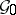is the real Banach subspace ofconsisting of transvections and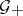is the subcone of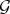such that for each,In other words,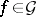admits a unique representation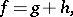(a25)

where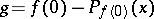is complete,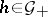and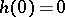.

The natural examples of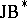triple systems are a complex Hilbert space, the space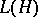of bounded linear operators on, and its subspacessuch thatif and only if(such subspaces are usually called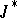-algebras). In the latter case the general form of transvections onis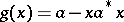, whereand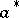is its conjugate. Thus, each semi-complete vector field on the open unit ball of a-algebra has the form(a26)

whereand.

In particular, when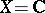is the complex plane and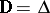, the open unit disc in, (a26) becomes(a27)

whereand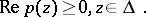(a28)

In 1978 E. Berkson and H. Porta [a4], solving an entirely different problem, gave a parametric representation of generators on the unit discin the complex plane. More precisely,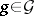if and only if for some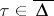,has the representation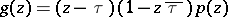with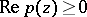everywhere. This pointis exactly the limit point of the semi-group generated by(that is, its Denjoy–Wolff point, cf. Denjoy–Wolff theorem). The Berkson–Porta formula has also been successfully exploited in other fields; for example, in the classical functional equations of E. Schröder and N.H. Abel (see [a5] and Functional equation; Schröder functional equation).

Letbe a complex Hilbert space with inner productand let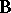be its open unit ball. Letdenote the Poincaré hyperbolic metric on[a7] (cf. also Poincaré model). A mappingis said to be-monotone if for each pairand positivethe following condition holds: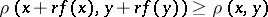wheneverandbelong to. It was shown in [a13] that ifis separable and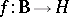is a bounded continuous mapping, thenis-monotone if and only if it generates a semi-group of-non-expansive self-mappings of. Note also that-monotonicity can be equivalently described as follows: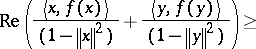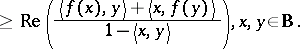For a bounded holomorphic mappingand for an arbitrarythe latter condition is a criterion forto be semi-complete. For the one-dimensional case, if, then this condition becomes the Berkson–Porta representation of semi-complete vector fields.

How to Cite This Entry:
Semi-group of holomorphic mappings. Encyclopedia of Mathematics. URL: http://encyclopediaofmath.org/index.php?title=Semi-group_of_holomorphic_mappings&oldid=51280
This article was adapted from an original article by Simeon ReichDavid Shoikhet (originator), which appeared in Encyclopedia of Mathematics - ISBN 1402006098. See original article